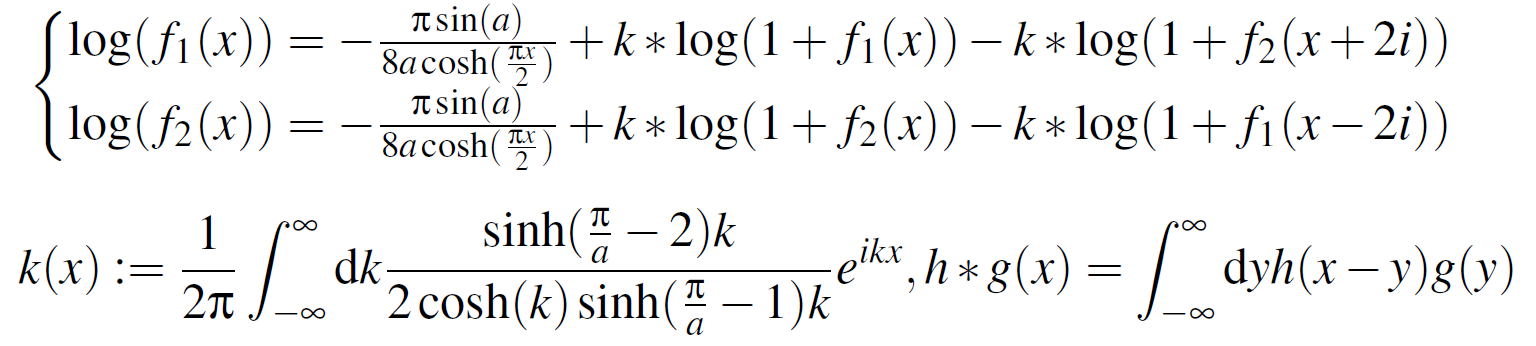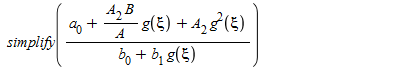## How to i solve the question B given in maple...## How do i solve this ordinary differential equation...## Hello, i want to ask can we convert matlab code to...

clc;clear;
% Define i1(t) and i2(t) as symbolic variable
syms i1(t) i2(t)

% Given differential equation
ode1 = diff(i1) == 0.5*i1 + -3*i2 +5*exp(-2*t);
ode2 = diff(i2) == 2*i1 - 6*i2;
odes = [ode1; ode2];

% Define initial conditions
cond1 = i1(0) == 1;
cond2 = i2(0) == -1;
conds = [cond1; cond2];

% Solution of system of differential equation
[i1(t), i2(t)] = dsolve(odes,conds)

fplot(i1(t))
hold on
fplot(i2(t))
legend('i1(t)','i2(t)')

Output:

i1(t) =
exp((t*(73^(1/2) - 11))/4)*(73^(1/2)/8 + 13/8)*((57*73^(1/2))/292 - exp((3*t)/4 - (73^(1/2)*t)/4)*((15*73^(1/2))/292 + 5/4) + 3/4) - exp(-(t*(73^(1/2) + 11))/4)*(73^(1/2)/8 - 13/8)*(exp((3*t)/4 + (73^(1/2)*t)/4)*((15*73^(1/2))/292 - 5/4) + (3*73^(1/2)*(73^(1/2) - 19))/292)

i2(t) =
exp(-(t*(73^(1/2) + 11))/4)*(exp((3*t)/4 + (73^(1/2)*t)/4)*((15*73^(1/2))/292 - 5/4) + (3*73^(1/2)*(73^(1/2) - 19))/292) + exp((t*(73^(1/2) - 11))/4)*((57*73^(1/2))/292 - exp((3*t)/4 - (73^(1/2)*t)/4)*((15*73^(1/2))/292 + 5/4) + 3/4)

## How do I solve numerically a set of non-linear int...

Hey everyone,

f_1 and f_2  are satisfying the set of non-linear integral equations I have attached to this message.
I know that I need to solve them numerically by iterations. Probably, the first guest of the function f_1 and f_2  is the driving term. a is just a parameter which can be fixed (I guess smaller than \pi/4). * is the convolution product and k is the momentum space parameter. I learnt that in order to solve them I should solve them in the Fourier space. I know also that I need to discretize these function in the “real ” space between {-L,+L} before applying the FFT or one of its relatives. Thank you for any suggestions or leads.## dsolve not working for N=1 or N=2....

Dear maple users.

Greetings for the day.

I hope you are all fine and safe.

In the below mention code, I need to plot "ax" at 0..1 when N=1 and N=2.

But the code only working for the N=0 case.

How to tackle this situation and plot the function for various values of "ax" at ax=0..1.

JBV.mw

Code:

restart;
PDEtools[declare](f(x), t(x), g(x), prime = (x));

N := 2; m := .2; pa := 3.14*(1/3); ax := ax; h2 := 1+.2*ax+.3*sin((2*3.14)*(ax-.2)); h1 := -1-.2*ax-.1*sin((2*3.14)*(ax-.2)+pa); a2 := 1.4+.1*sin((2*3.14)*(ax-.2))+.3*sin((2*3.14)*(ax-.2)+pa);

f(x):=sum(p^j*f[j](x),j=0..N);  t(x):=sum(p^j*t[j](x),j=0..N);

g(x):=sum(p^j*g[j](x),j=0..N);

Eq1 := (1-p)*(diff(f(x), $(x, 4)))+p*((1+.2)*(diff(f(x), $(x, 4)))-(.2*(1/3))*(diff((diff(f(x), $(x, 2)))^3, $(x, 2)))-2*(diff(f(x), $(x, 2)))+diff(t(x), $(x, 1))+diff(g(x), $(x, 1))); Eq2 := (1-p)*(1+1.2)*(diff(t(x), $(x, 2)))+p*((1+1.2)*(diff(t(x), $(x, 2)))+.1*(diff(t(x), $(x, 1)))*(diff(g(x), $(x, 1)))+.2*(diff(t(x), $(x, 1)))^2+.5*(diff(f(x), $(x, 1)))^2); Eq3 := (1-p)*(diff(g(x), $(x, 2)))+p*(diff(g(x), $(x, 2))+diff(t(x), $(x, 2)));

for j from 0 to N do
equ1[j] := coeff(Eq1, p, j) = 0;
equ2[j] := coeff(Eq2, p, j) = 0;
equ3[j] := coeff(Eq3, p, j) = 0;
end do;

con := f(h2) = (1/2)*a2, (D(f))(h2) = 0, f(h1) = -(1/2)*a2, (D(f))(h1) = 0; con := t(h2) = 1, t(h1) = 0; con := g(h2) = 1, g(h1) = 0;

for i to N do

con[i] := f[i](h2) = 0, (D(f[i]))(h2) = 0, f[i](h1) = 0, (D(f[i]))(h1) = 0; con[i] := t[i](h2) = 0, t[i](h1) = 0; con[i] := g[i](h2) = 0, g[i](h1) = 0 end do;

for i from 0 to N do
P:=dsolve({equ1[i],equ2[i],equ3[i],con[i],con[i],con[i]},{f[i](x),t[i](x),g[i](x)}):
f[i](x):=rhs(P);
t[i](x):=rhs(P);
g[i](x):=rhs(P);
end do:

f(x):=evalf(simplify(sum(f[n](x),n=0..N)));
Am := (1+.2)*(diff(f(x), \$(x, 3)));
with(plots);

display(plot(eval(Am, x = .6), ax = 0 .. 1, numpoints = 200, color = blue));

## How do i clear this error...

blessing.mw please i really need your help on this, i have been trying to fix this error for a while now.

## How to find triplets in Lie algebra at which Jacob...

Dear all

I have  Lie commutations for vectors e1, e2, e3, e4, e5, e6 as follow:

[e1, e3] = e3, [e1, e4] = e4, [e1, e5] = e5, [e1, e6] = e6, [e2, e3] = -e5, [e2, e4] = e6, [e3, e5] = e6

for which the command

Query("Jacobi")

returns the false result, which means, the vectors are not closed under Jacobi's identity. How can I find vector triplets for which Jacobi's identity does not hold?

## How do I solve this nonlinear system of Eqs. ?...

Hey everyone!
I have to solve this nonlinear system of equations . For small N (N=8), I tried to do it using solve but it is run forever. I have choosed \gamma to be equal to Pi/4.
test.mw## I need to debug the Error of parameters' must be s...

 > restart:
 > interface(rtablesize=10):
 > local gamma:local pi:
 > if false then  theta := 0.987: betae := 0.231: betay := 0.112: rho := 0.17: muh := 0.05 : sigma2 := 0.0411: sigma1 := 0.212: alpha1 := 0.111: alpha2 := 0.131 : eta := 0.134: thetaa := 0.7271: betaf := 0.00954: betah := 0.008220: gamma := 0.0012: mua := 0.0023: sigma3 := 0.203: d := 0.451: z := 0.072: end if:
 > # # D() is Maple's differential operator replated D(T) # with DD(T) in the following to avoid confusion #   ODE1 := diff(B(T), T) = theta-(betae*C(T)+betay*rho*G(T))*B(T)-muh*B(T)+sigma2*E(T):   ODE2 := diff(C(T), T) = (betae*C(T)+betay*rho*G(T))*B(T)-(muh+sigma1+alpha1)*C(T):   ODE3 := diff(E(T), T) = sigma1*C(T)-(muh+sigma2)*E(T):   ODE4 := diff(G(T), T) = alpha1*C(T)+alpha2*K(T)-eta*G(T):   ODE5 :=  diff(H(T), T) = thetaa-(betaf*H(T)+betah*gamma*G(T))*H(T)-mua*H(T)+sigma3*K(T):   ODE6 :=  diff(J(T), T) = (betaf*H(T)+betah*gamma*G(T))*H(T)-(mua+d+z+alpha2)*J(T):   ODE7 :=  diff(K(T), T) = z*J(T)-(mua+sigma3)*K(T):
 > if false then   B0 := 100: C0 := 60: E0 := 50: G0 := 55: H0 := 80: J0 := 80: K0 := 80:   end if:
 > # system + ic sys := { ODE1, ODE2, ODE3, ODE4, ODE5, ODE6, ODE7,                    B(0) = B0, C(0) = C0, E(0) = E0, G(0) = G0, H(0) = H0, J(0) = J0, K(0) = K0                  }: params := convert(indets(sys, name) minus {T}, list);(1)
 > # # Solve system #   ans := dsolve( { ODE1, ODE2, ODE3, ODE4, ODE5, ODE6, ODE7,                    B(0) = B0, C(0) = C0, E(0) = E0, G(0) = G0, H(0) = H0, J(0) = J0, K(0) = K0                                  },                  parameters = params,                  numeric                );
 >## Error, (in simplify/tools/_zn) too many levels of ...

Hello  i have this pb i don't now what to do :Error, (in simplify/tools/_zn) too many levels of recursion

thank you

## How do i correct the Plot matrix error of unexpect...

 >>(1)
 >(2)
 >>>## How do I plot a set of curves and a second funct...

I have a set of curves  :   plot({seq((6*x-2*t)/x^2, t = 1 .. 3)}, x = -1 .. 5, y = -1 .. 6)

and   a function           :   plot(3/x, x = 0 .. 5, y = -1 .. 6).

How is the calling sequence to plot the set and the function in the same graph ?